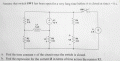# How to Find Equivalent Resistance and Current Across Resistor for RL Circuit?

Joined Dec 5, 2018
2
Hi everyone! I've never thought that in all my review with RC and RL circuits, I would come across one as difficult as this! So the problem asks me to find the time constant for the RL circuit when the switch closes at t = 0, and to find the current across the resistor R3 in terms of time. For the time constant, I attempted at redrawing the circuit at t = infinity to represent steady state and find the equivalent resistance from there. My experience with RL/RC circuits and circuit analysis has suggested to me that the inductor becomes a short circuit and that I can use superposition with the voltage source, and the current source to find Req. My understanding is that R3 is parallel to the series combination of R4 + R5, followed by R2 being in series with all of that. However, I do not know if this is completely correct. Part of me thinks that R1 is parallel to all of that, so when I attempted at doing this approach, I ended up with Req = 1.59 ohms, which for the time constant, led to tau = 0.000000630 seconds. I honestly think this is very likely wrong, but I could be wrong as well?

As for finding the current i(3) across the R3 resistor, I was thinking that if I find i3 at t<0 and t = infinity, both of which are at steady state, I can put them into a general solution equation, where i3(t) = i3(t=infinity) + [i3(t<0) - i3(t =infinity)] * e^(-t/tau). I tried using the mesh current method to find i3 for both cases, wherein the voltage source remained intact for t<0, and the current source only being present for t=infinity. I don't know if this was the right approach, but I did end up with i3(t<0) = 2.67 A and i3(t=infinity) = 1.78 A. It took a lot of re-drawing of the circuit, but I'm not sure whether this is a correct approach as well into finding i3(t)?

I've spent several hours nonstop trying to solve this problem, but I'm more surprised that I've been stuck for so long! Is there anyone that can help me with this, or point me in the right direction?Last edited by a moderator:

#### ericgibbs

Joined Jan 29, 2010
8,744
Hi Retro,
Welcome to AAC.
Please post your attempt at solving the problem so that we can guide you.
E
BTW

•Joined Dec 5, 2018
2

#### Attachments

• 1.6 MB Views: 2
Last edited by a moderator:

#### WBahn

Joined Mar 31, 2012
24,700
You know it's a first order circuit, right?

So you know the form of the equation after the switch closes. For the inductor current, you just need the initial current, the final current, and the time constant.

For the time constant, you need not the resistance seen by one of the supplies at infinite time, but the resistance as seen by the inductor at all time after the switch closes. Think Thevenin.